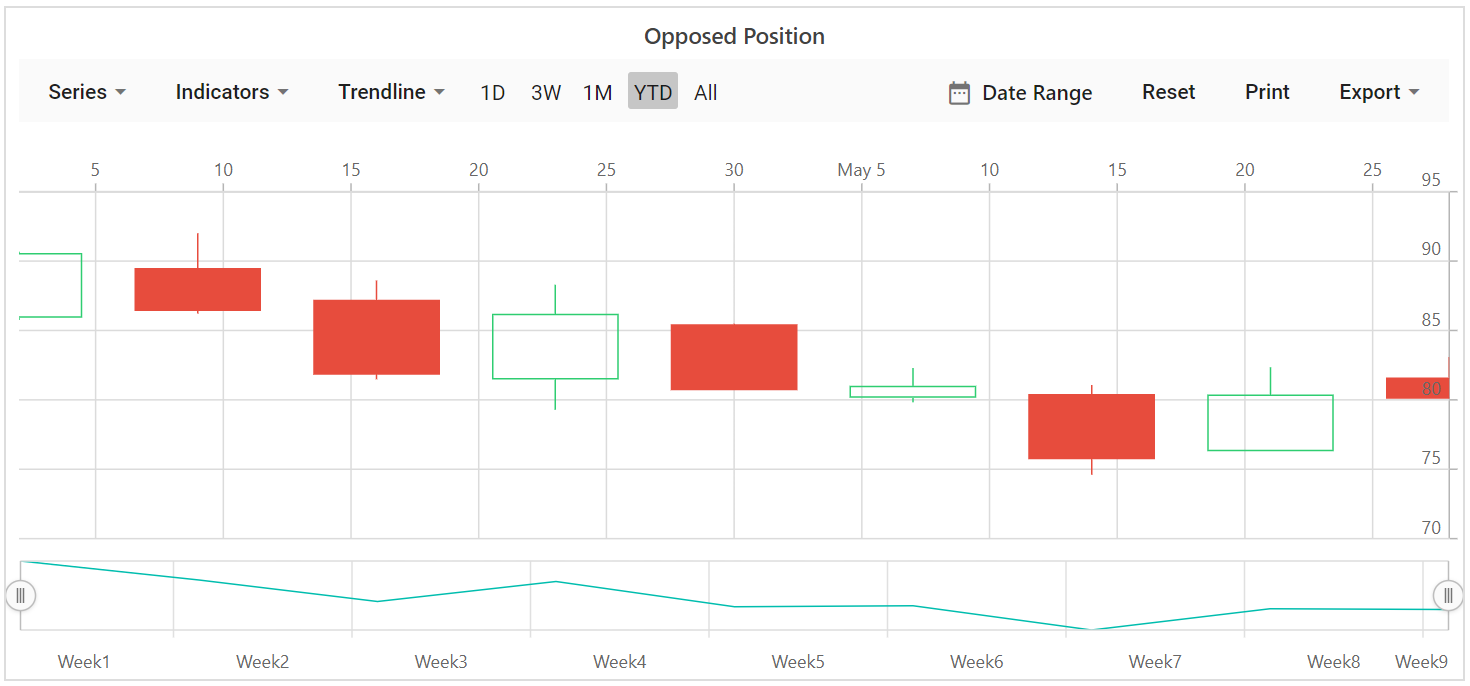Search results

# Axis Customization in Blazor Stock Chart component

28 Jul 2021 / 2 minutes to read

## Title

You can add a title to the axis using Title property to provide quick information to the user about the data plotted in the axis. Title style can be customized using `TitleStyle` property of the axis.

title.razor
``````@using Syncfusion.Blazor.Charts

<SfStockChart Title="AAPL Stock Price">
<StockChartSeriesCollection>
<StockChartSeries DataSource="@StockDetails" Type="ChartSeriesType.Line" XName="Date" YName="Y"></StockChartSeries>
</StockChartSeriesCollection>
</SfStockChart>

@code {

public class ChartData
{
public DateTime Date;
public Double Y;
}

public List<ChartData> StockDetails = new List<ChartData>
{
new ChartData { Date = new DateTime(2012, 04, 02), Y= 100},
new ChartData { Date = new DateTime(2012, 04, 09), Y= 10},
new ChartData { Date = new DateTime(2012, 04, 16), Y= 500},
new ChartData { Date = new DateTime(2012, 04, 23), Y= 80},
new ChartData { Date = new DateTime(2012, 04, 30), Y= 200},
new ChartData { Date = new DateTime(2012, 05, 07), Y= 600},
new ChartData { Date = new DateTime(2012, 05, 14), Y= 50},
new ChartData { Date = new DateTime(2012, 05, 21), Y= 700},
new ChartData { Date = new DateTime(2012, 05, 28), Y= 90}
};
}``````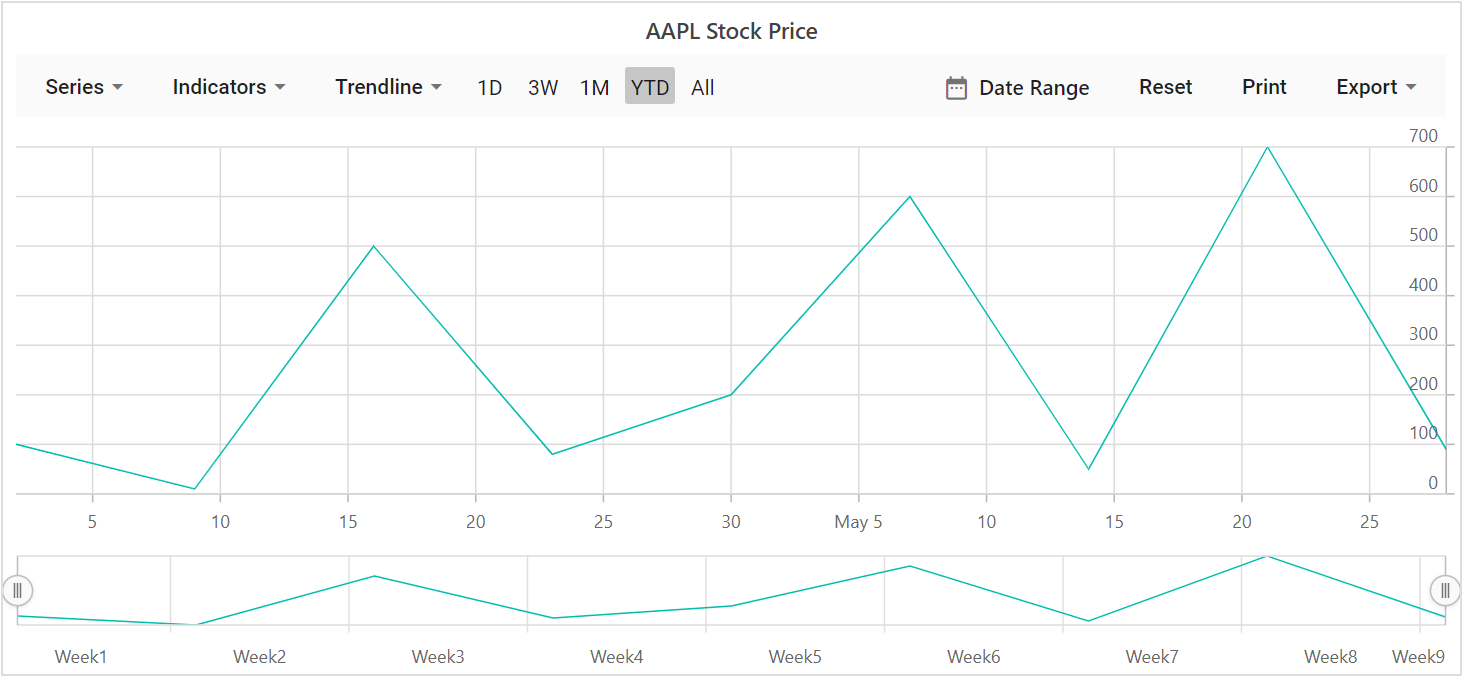## Tick Lines Customization

You can customize the  `Width``Color` and `Size` of the minor and major tick lines, using `MajorTickLines` and `MinorTickLines` properties in the axis.

tick.razor
``````@using Syncfusion.Blazor.Charts

<SfStockChart Title="AAPL Stock Price">
<StockChartPrimaryXAxis>
<StockChartAxisMajorTickLines Width="5" Color="blue"></StockChartAxisMajorTickLines>
<StockChartAxisMinorTickLines Width="0" Color="red"></StockChartAxisMinorTickLines>
</StockChartPrimaryXAxis>

<StockChartSeriesCollection>
<StockChartSeries DataSource="@StockDetails" Type="ChartSeriesType.Line" XName="Date" YName="Y"></StockChartSeries>
</StockChartSeriesCollection>
</SfStockChart>

@code {

public class ChartData
{
public DateTime Date;
public Double Y;
}

public List<ChartData> StockDetails = new List<ChartData>
{
new ChartData { Date = new DateTime(2012, 04, 02), Y= 100},
new ChartData { Date = new DateTime(2012, 04, 09), Y= 10},
new ChartData { Date = new DateTime(2012, 04, 16), Y= 500},
new ChartData { Date = new DateTime(2012, 04, 23), Y= 80},
new ChartData { Date = new DateTime(2012, 04, 30), Y= 200},
new ChartData { Date = new DateTime(2012, 05, 07), Y= 600},
new ChartData { Date = new DateTime(2012, 05, 14), Y= 50},
new ChartData { Date = new DateTime(2012, 05, 21), Y= 700},
new ChartData { Date = new DateTime(2012, 05, 28), Y= 90}
};
}``````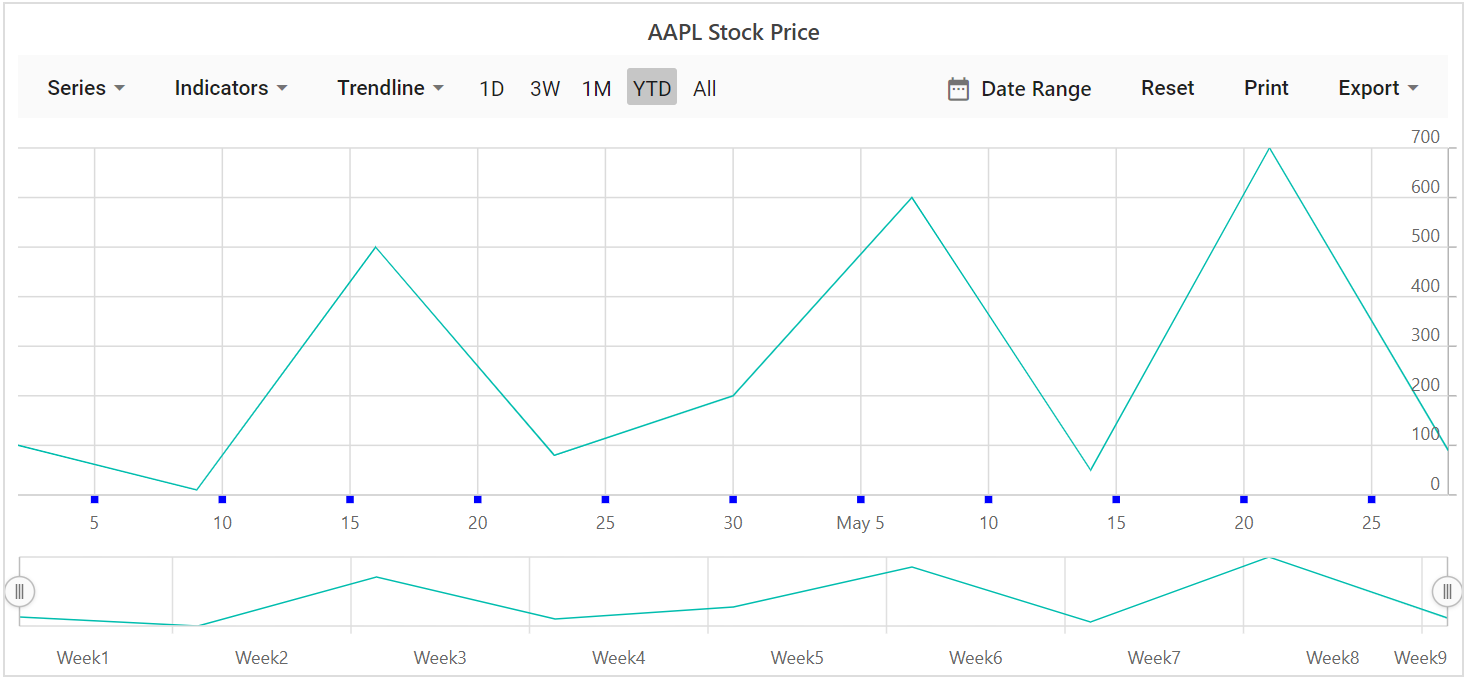## Grid Lines Customization

You can customize the  `Width``Color` and `DashArray` of the minor and major grid lines, using `MajorGridLines` and `MinorGridLines` properties in the axis.

grid.razor
``````@using Syncfusion.Blazor.Charts

<SfStockChart Title="AAPL Stock Price">
<StockChartPrimaryXAxis>
<StockChartAxisMajorGridLines Width="1" Color="blue"></StockChartAxisMajorGridLines>
<StockChartAxisMinorGridLines Width="0" Color="red"></StockChartAxisMinorGridLines>
</StockChartPrimaryXAxis>

<StockChartPrimaryYAxis>
<StockChartAxisMajorGridLines Width="1" Color="green"></StockChartAxisMajorGridLines>
<StockChartAxisMinorGridLines Width="0" Color="red"></StockChartAxisMinorGridLines>
</StockChartPrimaryYAxis>

<StockChartSeriesCollection>
<StockChartSeries DataSource="@StockDetails" Type="ChartSeriesType.Line" XName="Date" YName="Y"></StockChartSeries>
</StockChartSeriesCollection>
</SfStockChart>

@code {

public class ChartData
{
public DateTime Date;
public Double Y;
}

public List<ChartData> StockDetails = new List<ChartData>
{
new ChartData { Date = new DateTime(2012, 04, 02), Y= 100},
new ChartData { Date = new DateTime(2012, 04, 09), Y= 10},
new ChartData { Date = new DateTime(2012, 04, 16), Y= 500},
new ChartData { Date = new DateTime(2012, 04, 23), Y= 80},
new ChartData { Date = new DateTime(2012, 04, 30), Y= 200},
new ChartData { Date = new DateTime(2012, 05, 07), Y= 600},
new ChartData { Date = new DateTime(2012, 05, 14), Y= 50},
new ChartData { Date = new DateTime(2012, 05, 21), Y= 700},
new ChartData { Date = new DateTime(2012, 05, 28), Y= 90}
};
}``````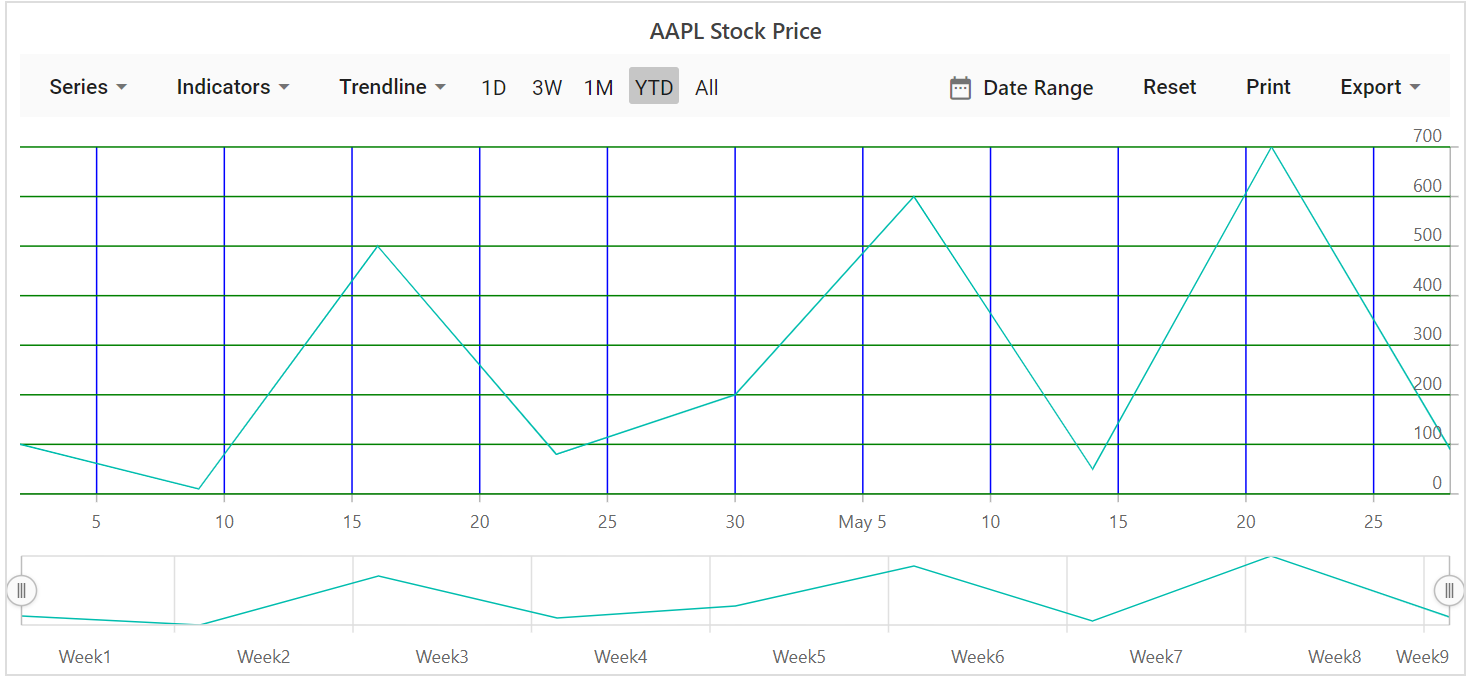## Multiple Axis

In addition to primary X and Y axis, we can add n number of axis to the chart. Series can be associated with this Axis, by mapping with axis’s unique name.

multiple.razor
``````@using Syncfusion.Blazor.Charts

<SfStockChart Title="Multiple Axis">
<StockChartAxes>
<StockChartAxis RowIndex="0" Name="yAxis"></StockChartAxis>
</StockChartAxes>

<StockChartPrimaryXAxis>
<StockChartAxisMajorGridLines Width="0"></StockChartAxisMajorGridLines>
</StockChartPrimaryXAxis>

<StockChartPrimaryYAxis Interval="40">
<StockChartAxisLineStyle Color="Transparent"></StockChartAxisLineStyle>
<StockChartAxisMajorTickLines Width="0" Color="Transparent"></StockChartAxisMajorTickLines>
</StockChartPrimaryYAxis>

<StockChartCrosshairSettings Enable="true"></StockChartCrosshairSettings>

<StockChartSeriesCollection>
<StockChartSeries DataSource="@StockDetails" Type="ChartSeriesType.Candle" XName="Date" High="High" Low="Low" Open="Open" Close="Close" Name="Apple"></StockChartSeries>
<StockChartSeries DataSource="@StockDetails" Type="ChartSeriesType.Column" YAxisName="yAxis" XName="Date" YName="Low" Name="Google"></StockChartSeries>
</StockChartSeriesCollection>
</SfStockChart>

@code {

public class ChartData
{
public DateTime Date;
public Double Open;
public Double Low;
public Double Close;
public Double High;
public Double Volume;
}

public List<ChartData> StockDetails = new List<ChartData>
{
new ChartData { Date = new DateTime(2012, 04, 02), Open= 85.9757, High = 90.6657,Low = 85.7685, Close = 90.5257,Volume = 660187068},
new ChartData { Date = new DateTime(2012, 04, 09), Open= 89.4471, High = 92,Low = 86.2157, Close = 86.4614,Volume = 912634864},
new ChartData { Date = new DateTime(2012, 04, 16), Open= 87.1514, High = 88.6071,Low = 81.4885, Close = 81.8543,Volume = 1221746066},
new ChartData { Date = new DateTime(2012, 04, 23), Open= 81.5157, High = 88.2857,Low = 79.2857, Close = 86.1428,Volume = 965935749},
new ChartData { Date = new DateTime(2012, 04, 30), Open= 85.4, High =  85.4857,Low = 80.7385, Close = 80.75,Volume = 615249365},
new ChartData { Date = new DateTime(2012, 05, 07), Open= 80.2143, High = 82.2685,Low = 79.8185, Close = 80.9585,Volume = 541742692},
new ChartData { Date = new DateTime(2012, 05, 14), Open= 80.3671, High = 81.0728,Low = 74.5971, Close = 75.7685,Volume = 708126233},
new ChartData { Date = new DateTime(2012, 05, 21), Open= 76.3571, High = 82.3571,Low = 76.2928, Close = 80.3271,Volume = 682076215},
new ChartData { Date = new DateTime(2012, 05, 28), Open= 81.5571, High = 83.0714,Low = 80.0743, Close = 80.1414,Volume = 480059584}
};
}``````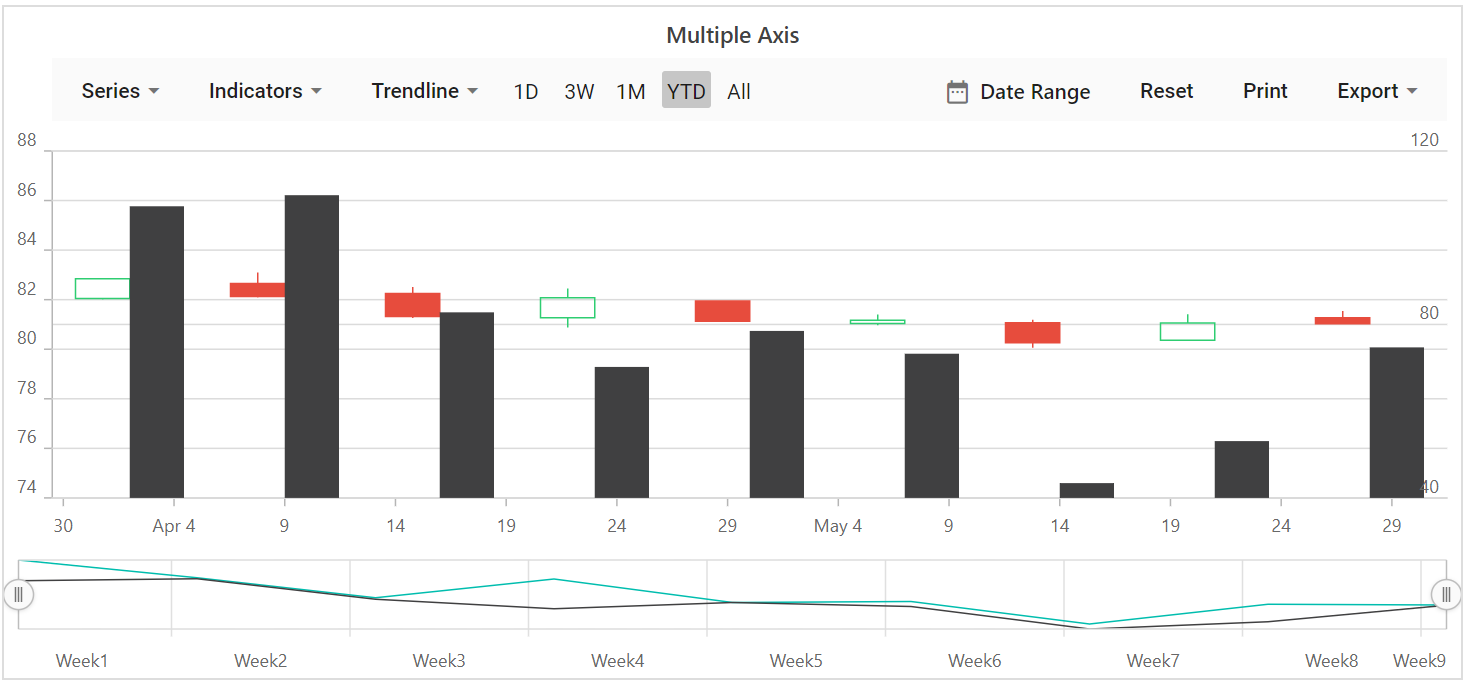## Inversed Axis

When an axis is inversed, highest value of the axis comes closer to origin and vice versa. To place an axis in inversed manner set this property `IsInversed` to true.

inversed.razor
``````@using Syncfusion.Blazor.Charts

<SfStockChart Title="Inversed Axis">
<StockChartPrimaryXAxis IsInversed="true"></StockChartPrimaryXAxis>

<StockChartSeriesCollection>
<StockChartSeries DataSource="@StockDetails" Type="ChartSeriesType.Candle" XName="Date" High="High" Low="Low" Open="Open" Close="Close" Name="Apple"></StockChartSeries>
</StockChartSeriesCollection>
</SfStockChart>

@code {

public class ChartData
{
public DateTime Date;
public Double Open;
public Double Low;
public Double Close;
public Double High;
public Double Volume;
}

public List<ChartData> StockDetails = new List<ChartData>
{
new ChartData { Date = new DateTime(2012, 04, 02), Open= 85.9757, High = 90.6657,Low = 85.7685, Close = 90.5257,Volume = 660187068},
new ChartData { Date = new DateTime(2012, 04, 09), Open= 89.4471, High = 92,Low = 86.2157, Close = 86.4614,Volume = 912634864},
new ChartData { Date = new DateTime(2012, 04, 16), Open= 87.1514, High = 88.6071,Low = 81.4885, Close = 81.8543,Volume = 1221746066},
new ChartData { Date = new DateTime(2012, 04, 23), Open= 81.5157, High = 88.2857,Low = 79.2857, Close = 86.1428,Volume = 965935749},
new ChartData { Date = new DateTime(2012, 04, 30), Open= 85.4, High =  85.4857,Low = 80.7385, Close = 80.75,Volume = 615249365},
new ChartData { Date = new DateTime(2012, 05, 07), Open= 80.2143, High = 82.2685,Low = 79.8185, Close = 80.9585,Volume = 541742692},
new ChartData { Date = new DateTime(2012, 05, 14), Open= 80.3671, High = 81.0728,Low = 74.5971, Close = 75.7685,Volume = 708126233},
new ChartData { Date = new DateTime(2012, 05, 21), Open= 76.3571, High = 82.3571,Low = 76.2928, Close = 80.3271,Volume = 682076215},
new ChartData { Date = new DateTime(2012, 05, 28), Open= 81.5571, High = 83.0714,Low = 80.0743, Close = 80.1414,Volume = 480059584}
};
}``````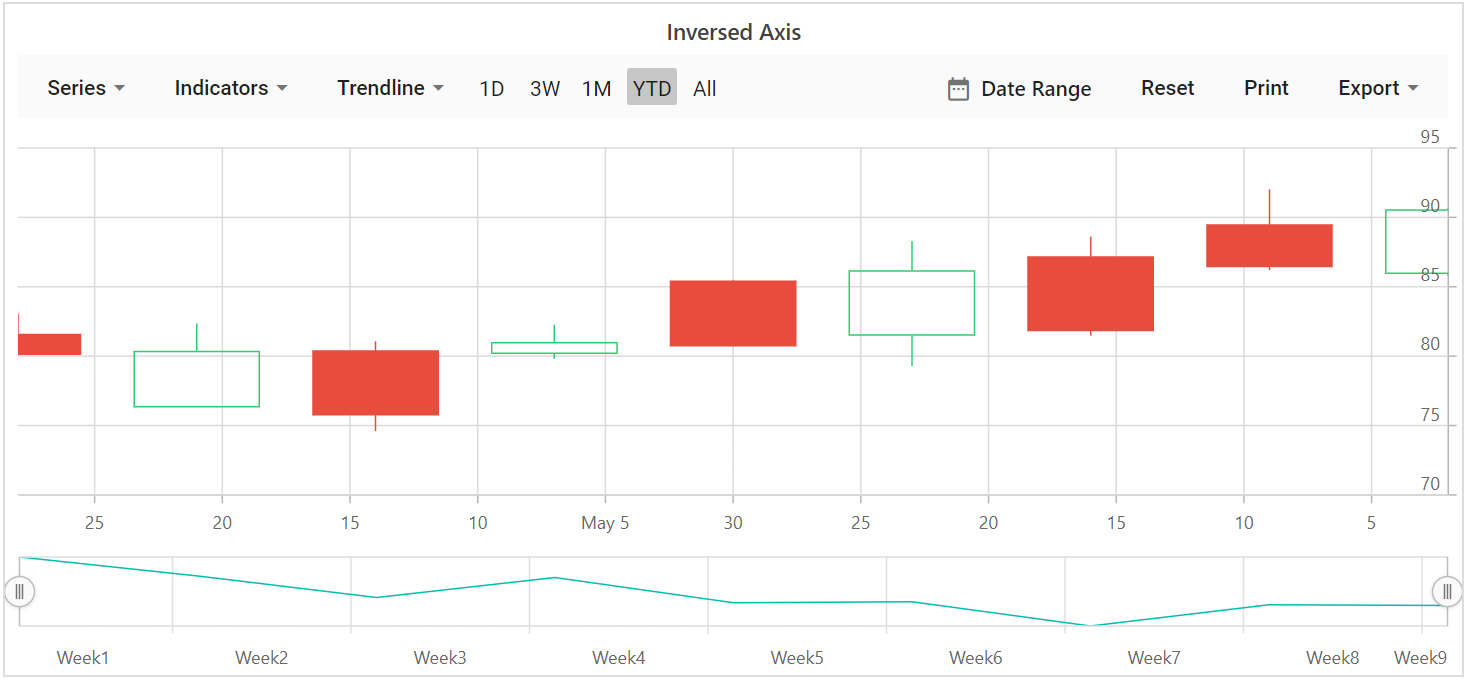## Opposed Position

To place an axis opposite from its original position, set `OpposedPosition` to true.

opposed.razor
``````@using Syncfusion.Blazor.Charts

<SfStockChart Title="AAPL Stock Price">
<StockChartPrimaryXAxis OpposedPosition="true"></StockChartPrimaryXAxis>

<StockChartSeriesCollection>
<StockChartSeries DataSource="@StockDetails" Type="ChartSeriesType.Line" XName="Date" High="High" Low="Low" Open="Open" Close="Close"></StockChartSeries>
</StockChartSeriesCollection>
</SfStockChart>

@code {

public class ChartData
{
public DateTime Date;
public Double Open;
public Double Low;
public Double Close;
public Double High;
public Double Volume;
}

public List<ChartData> StockDetails = new List<ChartData>
{
new ChartData { Date = new DateTime(2012, 04, 02), Open= 85.9757, High = 90.6657,Low = 85.7685, Close = 90.5257,Volume = 660187068},
new ChartData { Date = new DateTime(2012, 04, 09), Open= 89.4471, High = 92,Low = 86.2157, Close = 86.4614,Volume = 912634864},
new ChartData { Date = new DateTime(2012, 04, 16), Open= 87.1514, High = 88.6071,Low = 81.4885, Close = 81.8543,Volume = 1221746066},
new ChartData { Date = new DateTime(2012, 04, 23), Open= 81.5157, High = 88.2857,Low = 79.2857, Close = 86.1428,Volume = 965935749},
new ChartData { Date = new DateTime(2012, 04, 30), Open= 85.4, High =  85.4857,Low = 80.7385, Close = 80.75,Volume = 615249365},
new ChartData { Date = new DateTime(2012, 05, 07), Open= 80.2143, High = 82.2685,Low = 79.8185, Close = 80.9585,Volume = 541742692},
new ChartData { Date = new DateTime(2012, 05, 14), Open= 80.3671, High = 81.0728,Low = 74.5971, Close = 75.7685,Volume = 708126233},
new ChartData { Date = new DateTime(2012, 05, 21), Open= 76.3571, High = 82.3571,Low = 76.2928, Close = 80.3271,Volume = 682076215},
new ChartData { Date = new DateTime(2012, 05, 28), Open= 81.5571, High = 83.0714,Low = 80.0743, Close = 80.1414,Volume = 480059584}
};
}``````